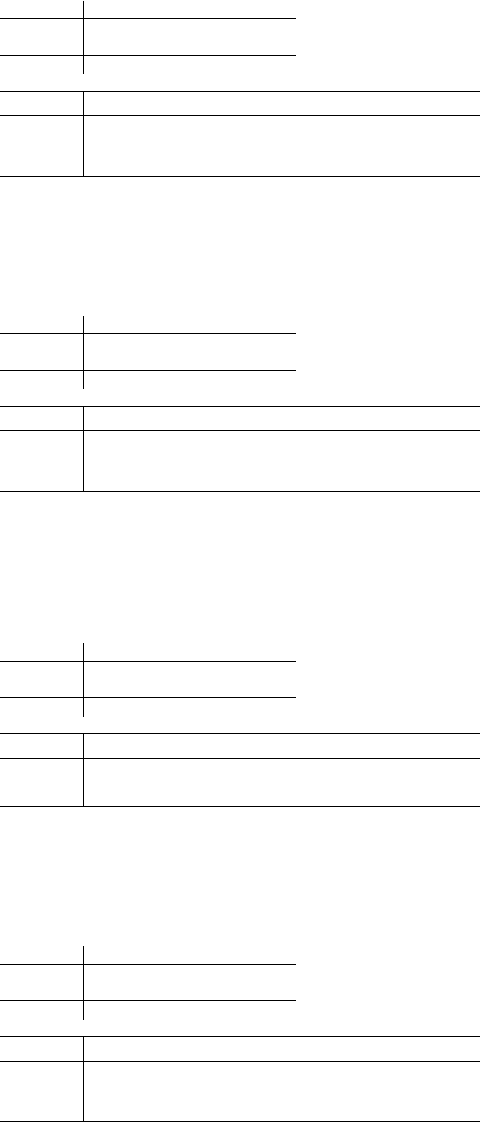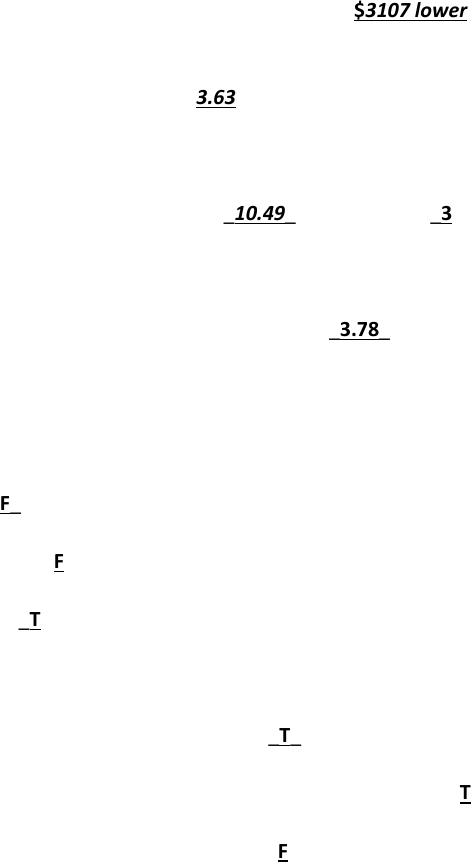# ECO 311 Miami U eco311Finalfall17 key

18 views9 pages
Eco311, Final Exam, Fall 2017
Prof. Bill Even
Directions
Each question is worth 4 points unless indicated otherwise.
Place all answers in the space provided below or within each question. Round all numerical answers to the nearest
100th (e.g. 1.23) unless told otherwise.
Unlock document

This preview shows pages 1-3 of the document.
Unlock all 9 pages and 3 million more documents.Eco311, Final Exam, Fall 2017
Prof. Bill Even
Using a sample of people aged 66 and above drawn from the Current Population Survey, I estimated a regression of annual social
security income measured in 1000s of \$ (SSINC) on a person’s age, years of education, and a dummy variable indicating whether the
person is female and followed this regression with several supplemental regressions.
Prob > F = 0.0000
F( 3, 17474) = 1.2e+06
( 3) female = 0
( 2) female - school = 0
( 1) female - age = 0
. test female=age=school=0
_cons 138.9225 .366929 378.61 0.000 138.2032 139.6417
school 21.37386 .0205686 1039.15 0.000 21.33354 21.41417
age .27867 .0046919 59.39 0.000 .2694733 .2878666
female -85.07383 .0559569 -1520.35 0.000 -85.18351 -84.96415
y2 Coef. Std. Err. t P>|t| [95% Conf. Interval]
Total 48864118.9 17,477 2795.90999 Root MSE = 3.6436
Residual 231976.883 17,474 13.2755455 R-squared = 0.9953
Model 48632142 3 16210714 Prob > F = 0.0000
F(3, 17474) > 99999.00
Source SS df MS Number of obs = 17,478
. reg y2 female age school
Prob > F = 0.0228
F( 2, 17475) = 3.78
( 2) y = 0
( 1) y - y2 = 0
. test y=y2=0
_cons -50.89931 64.17269 -0.79 0.428 -176.6842 74.88556
y2 -.4066632 .3462225 -1.17 0.240 -1.085294 .2719675
y 12.76254 9.500862 1.34 0.179 -5.860093 31.38518
u2 Coef. Std. Err. t P>|t| [95% Conf. Interval]
Total 486857193 17,477 27857.0231 Root MSE = 166.88
Residual 486646440 17,475 27848.151 R-squared = 0.0004
Model 210753.086 2 105376.543 Prob > F = 0.0228
F(2, 17475) = 3.78
Source SS df MS Number of obs = 17,478
. reg u2 y y2
Prob > F = 0.0124
F( 3, 17474) = 3.63
( 3) female = 0
( 2) female - school = 0
( 1) female - age = 0
. test female=age=school=0
_cons 50.36331 16.80449 3.00 0.003 17.42484 83.30178
school 2.742589 .9419935 2.91 0.004 .896188 4.588991
age -.17304 .2148801 -0.81 0.421 -.5942264 .2481465
female -1.903972 2.562695 -0.74 0.458 -6.927109 3.119165
u2 Coef. Std. Err. t P>|t| [95% Conf. Interval]
Total 486857193 17,477 27857.0231 Root MSE = 166.87
Residual 486554144 17,474 27844.4629 R-squared = 0.0006
Model 303048.234 3 101016.078 Prob > F = 0.0124
F(3, 17474) = 3.63
Source SS df MS Number of obs = 17,478
. reg u2 female age school
. gen y2=y^2
. gen u2=u^2
. predict y, xb
. predict u, residual
_cons 11.84683 .6801095 17.42 0.000 10.51375 13.17991
school .7802276 .0381243 20.47 0.000 .7055002 .854955
age .0093096 .0086966 1.07 0.284 -.0077366 .0263559
female -3.107464 .1037171 -29.96 0.000 -3.31076 -2.904168
ssinc Coef. Std. Err. t P>|t| [95% Conf. Interval]
Total 861852.969 17,477 49.3135532 Root MSE = 6.7534
Residual 796963.424 17,474 45.6085283 R-squared = 0.0753
Model 64889.5451 3 21629.8484 Prob > F = 0.0000
F(3, 17474) = 474.25
Source SS df MS Number of obs = 17,478
. reg ssinc female age school
Unlock document

This preview shows pages 1-3 of the document.
Unlock all 9 pages and 3 million more documents.Eco311, Final Exam, Fall 2017
Prof. Bill Even
Use the regressions from the prior page to answer the following questions.
1. Holding education and age constant, women’s average social security benefits are \$3107 lower than men’s.
2. The f-statistic for the Breusch-Pagan test of heteroscedasticity is 3.63_____ and implies that the null hypothesis
of homoscedasticity (should, should not) be rejected at the .01 level of significance.
3. The LM statistic for the Breusch-Pagan test for heteroscedasticity is _10.49______ and has _3_ degrees of
freedom.
4. The f-statistic for the simple version of the White test for heteroscedasticity is __3.78____ and implies that that
null hypothesis of homoscedasticity (should, should not) be rejected at the .05 level of significance.
5. Based on the regressions presented, indicate whether each of the following is true (T) or false (F). The variance
of the residual from the social security regression is
a. Greater for women than men. __F___
b. Greater for older than younger people. _F____
c. Greater for more educated people. __T__
6. If the null hypothesis of homoscedasticity is rejected, indicate whether each of the following is true (T) or false
(F)
a. The standard OLS estimates of the coefficients remain unbiased _T___
b. The standard OLS estimates of the standard errors for the coefficients are incorrect __T___
c. The standard OLS estimates of the coefficients remain efficient __F__
7. Suppose you have data on expenditures per pupil by school district and you estimate the following regression
=0+1+2+
Where the subscript i indexes the school district, expendpp is expenditures per pupil, income is average family
income, and married is the percent of households with married couples. The residuals from this regression (u)
are likely to be
a. Heteroskedastic and their variance will be greater in districts with larger populations
b. Homoskedastic and their variance will be greater districts with larger populations
c. Heteroskedastic and their variance will be greater in districts with smaller populations.
d. Homoskedastic and their variance will be greater districts with smaller populations
Unlock document

This preview shows pages 1-3 of the document.
Unlock all 9 pages and 3 million more documents.